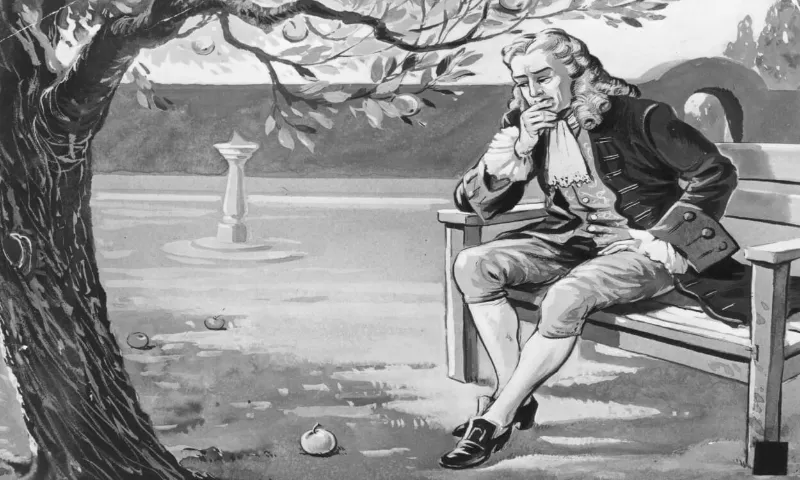### Sir Isaac Newton

Sir Isaac Newton was 23 when he discovered the law of gravity.Share

Sir Isaac Newton was an English mathematician, physicist, astronomer, theologian, and author (described in his own day as a "natural philosopher") who is widely recognized as one of the most influential scientists of all time, and a key figure in the scientific revolution. His book Philosophiæ Naturalis Principia Mathematica (Mathematical Principles of Natural Philosophy), first published in 1687, laid the foundations of classical mechanics. Newton also made seminal contributions to optics and shares credit with Gottfried Wilhelm Leibniz for developing the infinitesimal calculus.

In Principia, Newton formulated the laws of motion and universal gravitation that formed the dominant scientific viewpoint until it was superseded by the theory of relativity. Newton used his mathematical description of gravity to prove Kepler's laws of planetary motion, account for tides, the trajectories of comets, the precession of the equinoxes, and other phenomena, eradicating doubt about the Solar System's heliocentricity.

He demonstrated that the motion of objects on Earth and celestial bodies could be accounted for by the same principles. Newton's inference that the Earth is an oblate spheroid was later confirmed by the geodetic measurements of Maupertuis, La Condamine, and others, convincing most European scientists of the superiority of Newtonian mechanics over earlier systems.

Newton built the first practical reflecting telescope and developed a sophisticated theory of color based on the observation that a prism separates white light into the colors of the visible spectrum. His work on light was collected in his highly influential book Opticks, published in 1704. He also formulated an empirical law of cooling, made the first theoretical calculation of the speed of sound, and introduced the notion of a Newtonian fluid. In addition to his work on calculus, as a mathematician, Newton contributed to the study of power series, generalized the binomial theorem to non-integer exponents, developed a method for approximating the roots of a function, and classified most of the cubic plane curves.

Newton was a fellow of Trinity College and the second Lucasian Professor of Mathematics at the University of Cambridge. He was a devout but unorthodox Christian who privately rejected the doctrine of the Trinity. Unusually for a member of the Cambridge faculty of the day, he refused to take holy orders in the Church of England. Beyond his work on the mathematical sciences, Newton dedicated much of his time to the study of alchemy and biblical chronology, but most of his work in those areas remained unpublished until long after his death.The Internet was originally called ARPANet (Advanced Research Projects Agency Network) designed by the US Department of Defense.